### Home > CC1MN > Chapter 7 Unit 7 > Lesson CC1: 7.3.1 > Problem7-87

7-87.

Draw the table below on your paper and look carefully at the algebra tiles to fill in each of the steps. Use your own numbers in the trials, again considering fractions, decimals, and zero.

 Steps Trial 1 Trial 2 Trial 3 Algebra Tile Picture 1. Pick a number.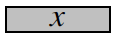2. Add ____.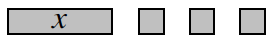3. Multiply by ____.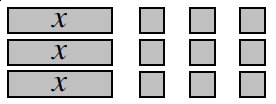4. Subtract ____.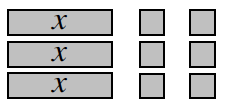5. Divide by ____.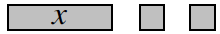6. Subtract the original number.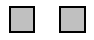Notice how the tiles increase or decrease after each step.

By how much did the algebra tiles increase in Step 2?

By how much was that number multiplied in Step 3?
Now complete the rest of the steps using this method.

Substitute in your own numbers for each trial.

 Steps Trial 1 Trial 2 Trial 3 Algebra Tile Picture 1. Pick a number.2. Add $\color{red}3$.3. Multiply by $\color{red}3$.4. Subtract $\color{red}3$.5. Divide by $\color{red}3$.6. Subtract the original number.Steps Trial 1 Trial 2 Trial 3 Algebra Tile Picture 1. Pick a number.2. Add $\color{red}3$.3. Multiply by ____ .4. Subtract ____.5. Divide by ____.6. Subtract the original number.Steps Trial 1 Trial 2 Trial 3 Algebra Tile Picture 1. Pick a number.2. Add $\color{red}3$.3. Multiply by $\color{red}3$.4. Subtract ____.5. Divide by ____.6. Subtract the original number.Steps Trial 1 Trial 2 Trial 3 Algebra Tile Picture 1. Pick a number.2. Add $\color{red}3$.3. Multiply by $\color{red}3$.4. Subtract $\color{red}3$.5. Divide by $\color{red}3$.6. Subtract the original number.Steps Trial 1 Trial 2 Trial 3 Algebra Tile Picture 1. Pick a number. $\color{purple}3$2. Add $\color{red}3$. $\color{purple}6$3. Multiply by $\color{red}3$. $\color{purple}{18}$4. Subtract $\color{red}3$. $\color{purple}{15}$5. Divide by $\color{red}3$. $\color{purple}{5}$6. Subtract the original number. $\color{purple}{2}$